# Axioms of Probability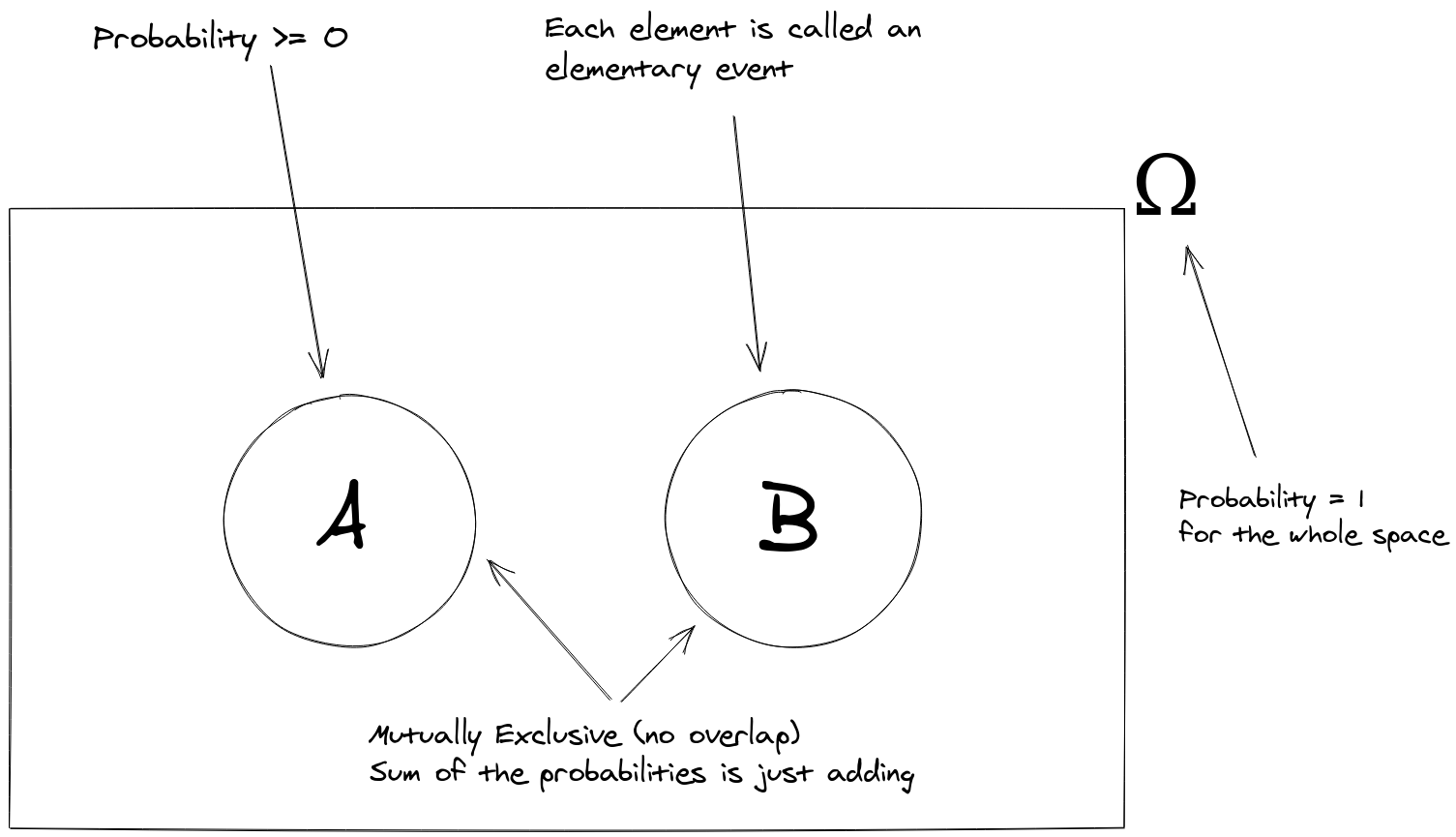This then leads to further rules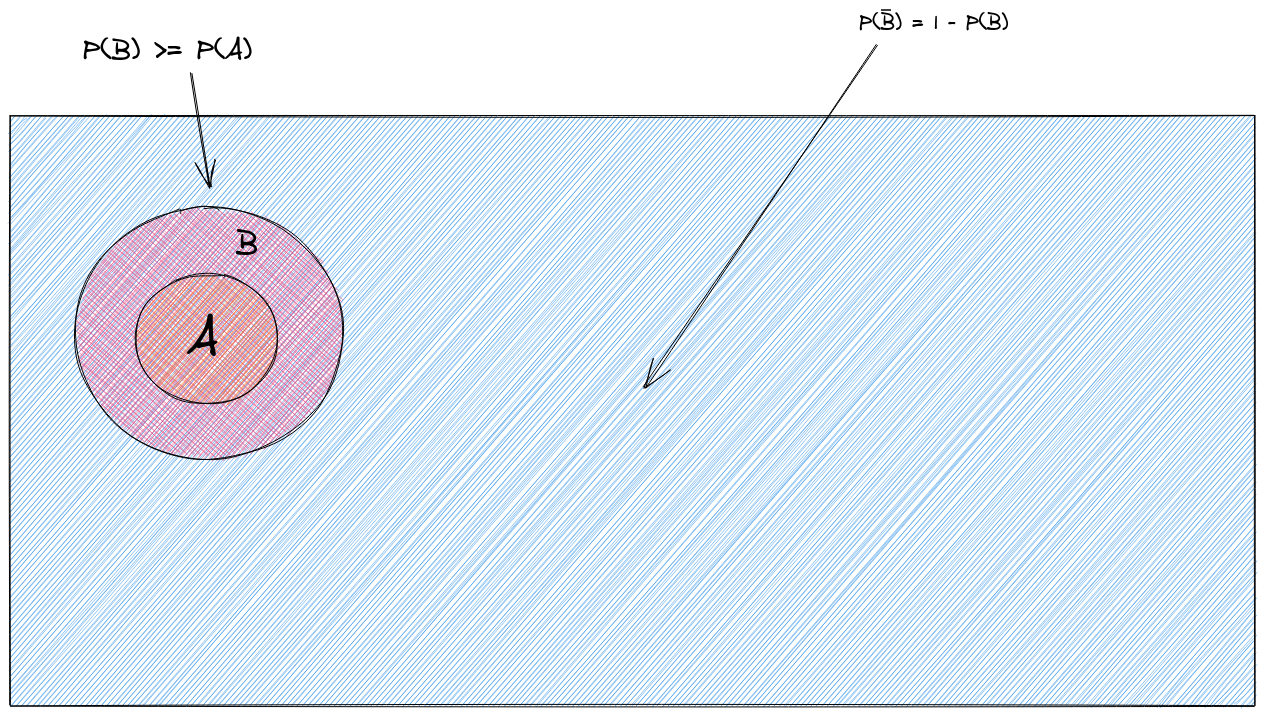# Conditional Probability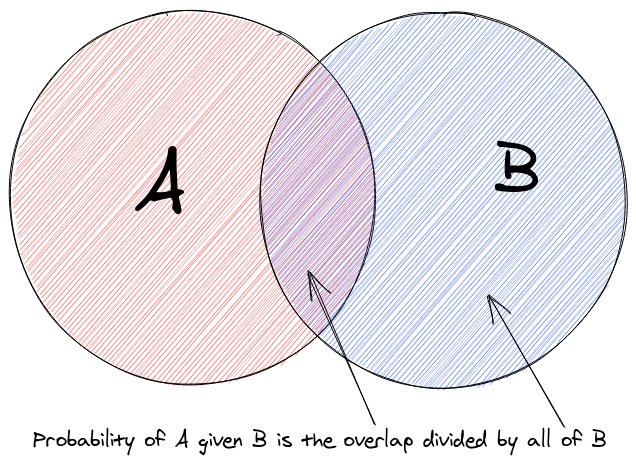# Uniform Distribution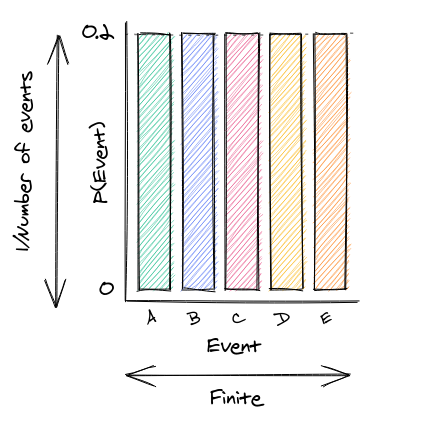# Independent Probabilities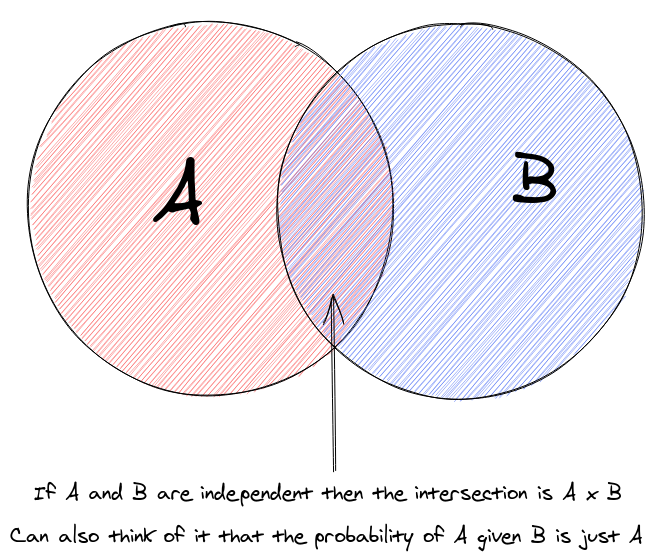## Pairwise Independence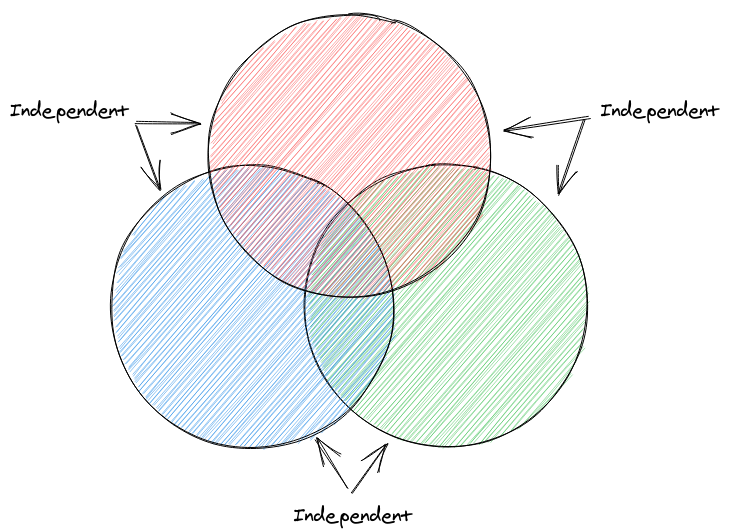Every pair of events are independent of each other

## Mutual Independence

The probability of all of a subset of events happening in the multiplication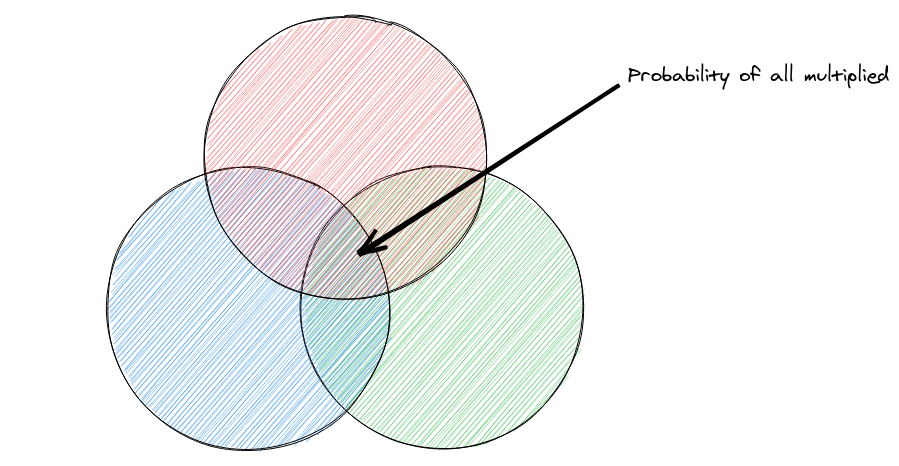# Random variable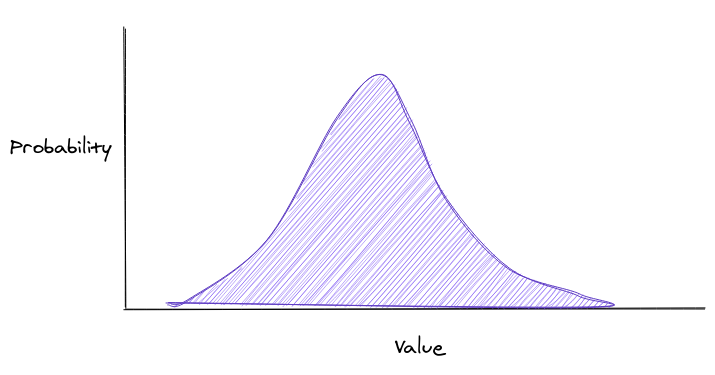The probability that a variable takes a certain value

## Independence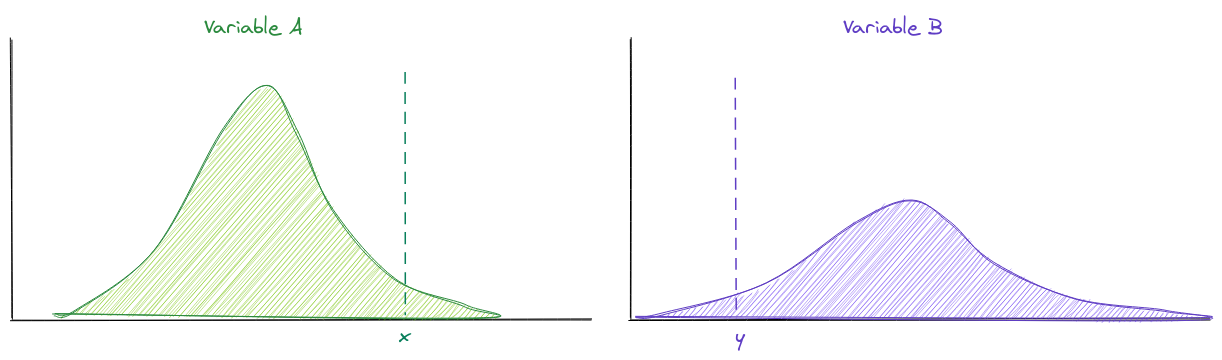The probability \$A=x\$ and \$B=y\$ is the probability \$A=x\$ multiplied by the probability \$B=y\$

## Expected value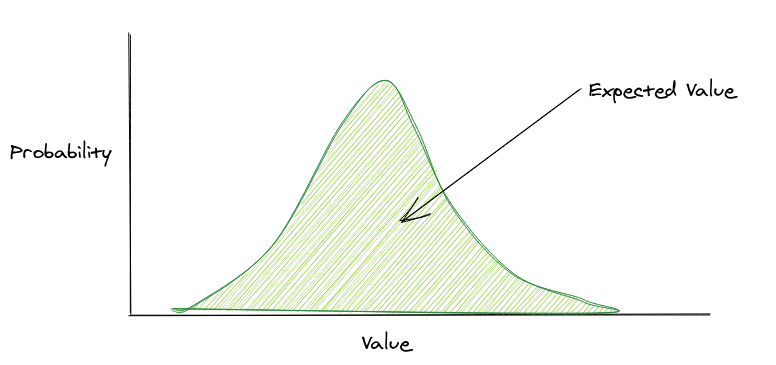The expected value is x times the probability of x for all x

This can also be shown as the area under the curve

## Linearity of expectation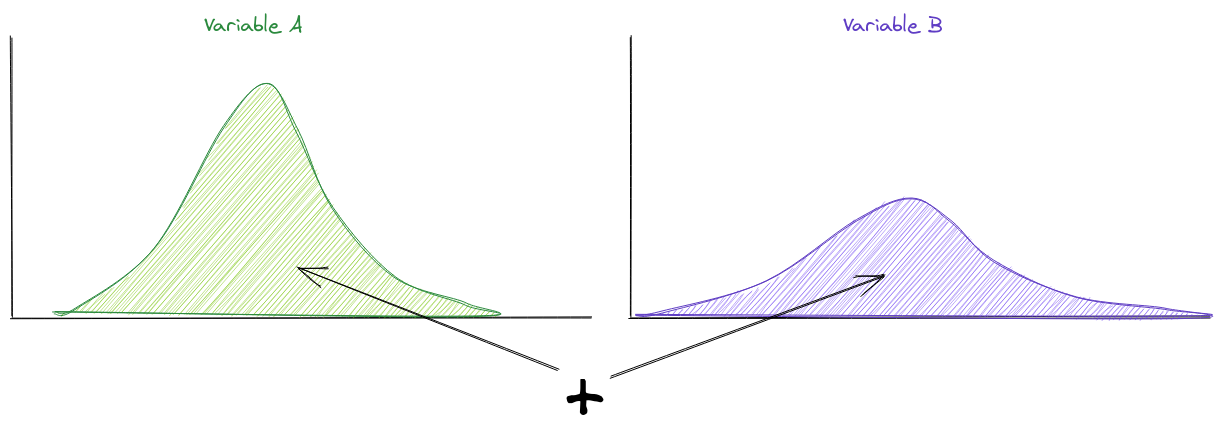The expected value of A and B is the expected value of A plus the expected value of B

This doesn’t apply for multiplication, only if they are independent